# Graphical Representation of Motion## Graphical Representation of Motion

• A graph is a pictorial representation of the relation between two sets of data of which one set is of dependent variables and the other set is of independent variables.
• To describe the motion of an object, we can use line graphs. In this case, line graphs show dependence of one physical quantity, such as distance or velocity, on another quantity, such as time.

### Distance Time Graphs

• The change in the position of an object with time can be represented on the distance-time graph.
• In this graph, time is taken along the x-axis and distance is taken along the y-axis.
• Distance time graphs of a moving body can be used to calculate the speed of the body as they specifically represent velocity.
• The distance time graph for a body moving at uniform speed is always a straight line as distance travelled by the body is directly proportional to time as shown below in the figure 1.
• The distance time graph for a body moving with non uniform speed is a curve and is shown below in the figure 2.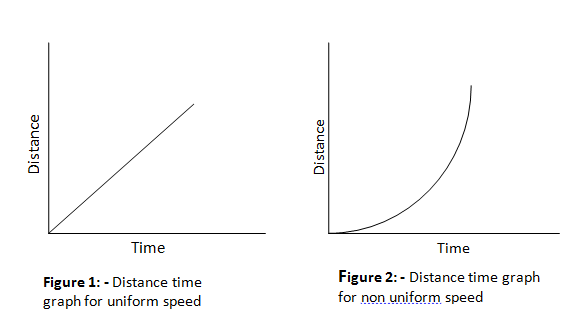• The distance time graph is parallel to time axis when the object is at rest and is shown below in figure 3.• To calculate speed of the body from distance time graph say at point A first draw a perpendicular AB on time axis and a perpendicular ACAC on distance axis so that ABAB represents the distance travelled by the body in time interval OB and since we know that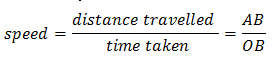### Velocity time graphs

• The variation in velocity with time for an object moving in a straight line can be represented by a velocity-time graph.
• In this graph, time is represented along the x-axis and the velocity is represented along the y-axis.
• The product of velocity and time give displacement of an object moving with uniform velocity. The area enclosed by velocity-time graph and the time axis will be equal to the magnitude of the displacement.
• If a body moves with a constant velocity then velocity time graph for this body would be straight line parallel to time axis as shown below in the figure 5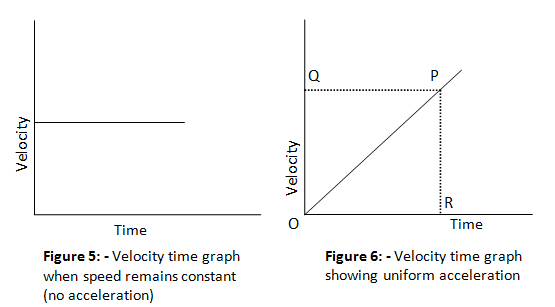• The velocity time graph of uniformly changing velocity is shown in figure 6 and is a straight line. We can find out the value of acceleration using the velocity time graph.
• For calculating acceleration at time corresponding to point R draw a perpendicular RP from point R as shown in figure 6 and we know that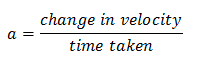Here change in velocity is represented by PR and time taken is equal to OR. So,which is equal to the slope of velocity time graph. So we conclude that slope of velocity time graph of moving body gives its acceleration.
• The distance travelled by moving body in a given time will be equal to area of triangle OPR as shown in figure 6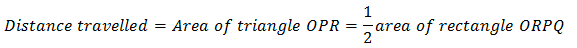so,• When the velocity of a body changes in an irregular manner then velocity time graph of the body is a curved line.

Don't miss out!